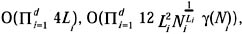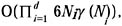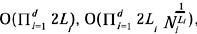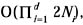# DATA CUBES FOR OTHER AGGREGATE OPERATORS

Popular aggregate operators like MIN and MAX cannot be handled by the techniques discussed in the previous section due to the lack of an inverse operation. This section summarizes approaches that work for these two operators as well. Some of them take advantage of specific monotonicity properties, while others apply whenever the domain of the measure attribute forms a commutative semi-group under the aggregate operator.

In Ho et al. (1997), a technique for the computation of range max queries is proposed. The data cube is recursively partitioned into smaller hyper-rectangular chunks (similar to a multidimensional quad tree). For each chunk the position of the maximum value in the chunk is stored. The corresponding tree is traversed top-down, using pruning techniques to reduce the number of accesses. Lee, Ling, & Li (2000) use a similar data structure but a different pruning strategy. They obtain constant average query cost and logarithmic update cost in the size of the data cube (for fixed dimensionality).

Poon (2001) proposes a general approach for commutative semi-groups. Similar to iterative data cubes, Poon's approach combines one-dimensional schemes to obtain a multidimensional pre-aggregation technique. Query, update, and storage costs areandrespectively. Here Li {l,, log Ni} denotes a user-controlled parameter and γ(Ni) a slow-growing function. Applied to invertible operators the query, update, andandrespectively. Note, however, that techniques like IDC—which are optimized to take advantage of the inverse operator—achieve better cost tradeoffs for invertible operators. For instance, for Li=1, an IDC that uses PS in each dimension achieves the same query but lower update and storage costs (cf., Table 1). Similarly IDC using RPS (DDC) in each dimension offers a better solution than the above technique for Li = 2 (Li = log Ni).Multidimensional Databases: Problems and Solutions
ISBN: 1591400538
EAN: 2147483647
Year: 2003
Pages: 150